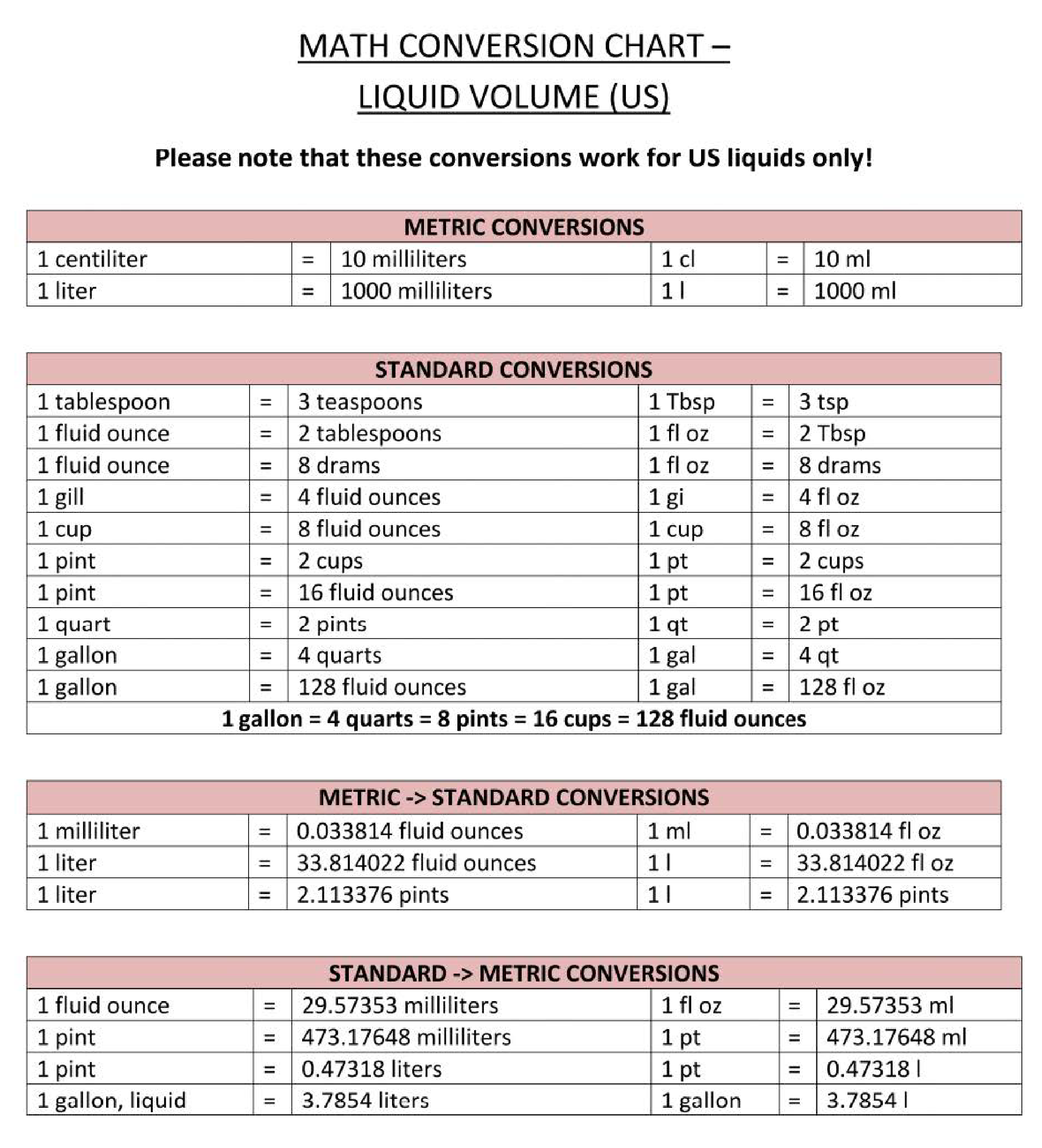# Liquid volume conversion chartCliquez sur l'image pour zoomer / Cliquez sur le bouton ci-dessous pour voir plus d'images

## Enregistrer, Remplir les champs vides, Imprimer, Terminer!How to convert liquid volume into different metrics? Download this liquid volume conversion reference chart template that will perfectly suit your needs!

Formats de fichiers gratuits disponibles:

.pdf

• Ce document a été certifié par un professionnel
• 100% personnalisable

Évaluation du modèle: 8

Aucun Malware/Virus trouvé, scanné par:Life Privé Education Éducation math mathematics mathématiques Conversion Chart Tableau de conversion volume conversion chart tableau de conversion de volume math conversion chart tableau de conversion mathématique math conversions chart tableau de conversions mathématiques maths conversion table pdf table de conversion maths pdf mathematical conversions list liste de conversions mathématiques mathematical units conversion table pdf table de conversion d'unités mathématiques pdf math conversion calculator calculatrice de conversion mathématique math conversion formulas formules de conversion mathématique unit conversion chart printable tableau de conversion d'unités imprimable basic metric conversions chart tableau des conversions métriques de base metric conversion chart math templates for kindergarten math templates for teachers math templates free download math themed template math worksheet mathematics certificates mathematics formulas math templates maths templates free microsoft powerpoint templates for mathematics microsoft word math templates powerpoint math templates unit conversion chart pdf unit conversion calculator conversion of units of measurement unit conversion chart physics unit conversion chart chemistry unit conversion chart unit conversion chart pdf conversion calculator unit converter unit conversion chart download unit conversion chart chemistry metric conversion physics all unit conversion chart unit conversion chart sample unit conversion chart template chemistry metric conversion chart physics unit conversion chart

How to convert liquid volume into different metrics? Download this liquid volume conversion reference chart template that will perfectly suit your needs!

Conversions are often necessary and common in many industries. From cooking to physics... Often, conversion is needed between units used in the metric system and the imperial system. Therefore, are common online calculators or offline metric conversion charts available.

This Metric conversion chart aims to help to convert units of measurement with Metric Converter and Metric Conversion Table into Imperial system units and to better understand alternative measurements that they are unfamiliar with. Therefore, we support you by providing this liquid volume conversion chart template, which will save your time and effort and help you to reach the next level of success in your studies, work, or business!

Liquid volume Conversion Chart, shows:
• metric liquid volume conversions;
• standard liquid volume conversions;
• imperial liquid volume conversions;
• metric to standard conversions;
• standard to metric conversions.

This Liquid volume Conversion Chart is intuitive, ready-to-use, and structured in a smart way. Try it now and let this mathematics template inspire you. We certainly encourage you to use this Liquid volume Conversion Chart for your own benefit and are confident it will fit your needs

Download this Liquid volume Conversion Chart now and print and place it in sight!

AVERTISSEMENT
Rien sur ce site ne doit être considéré comme un avis juridique et aucune relation avocat-client n'est établie.

Si vous avez des questions ou des commentaires, n'hésitez pas à les poster ci-dessous.Voir plus